# RD Sharma Solutions for Class 9 Maths Chapter 16 Circles Exercise 16.5

The RD Sharma Class 9 solutions for the chapter “Circle” exercise 16.5 is given here. In this exercise, students will learn about cyclic quadrilateral. A quadrilateral is a cyclic quadrilateral if its all vertices lie on a circle. This exercise includes several questions to help the students learn more about circles. Students are advised to click on the link below and get pdf to practice more on topic – circle. RD Sharma Solutions class 9 chapter 16 to help students in their endeavor to have a better understanding of circles.

## Download PDF of RD Sharma Solutions for Class 9 Maths Chapter 16 Circles Exercise 16.5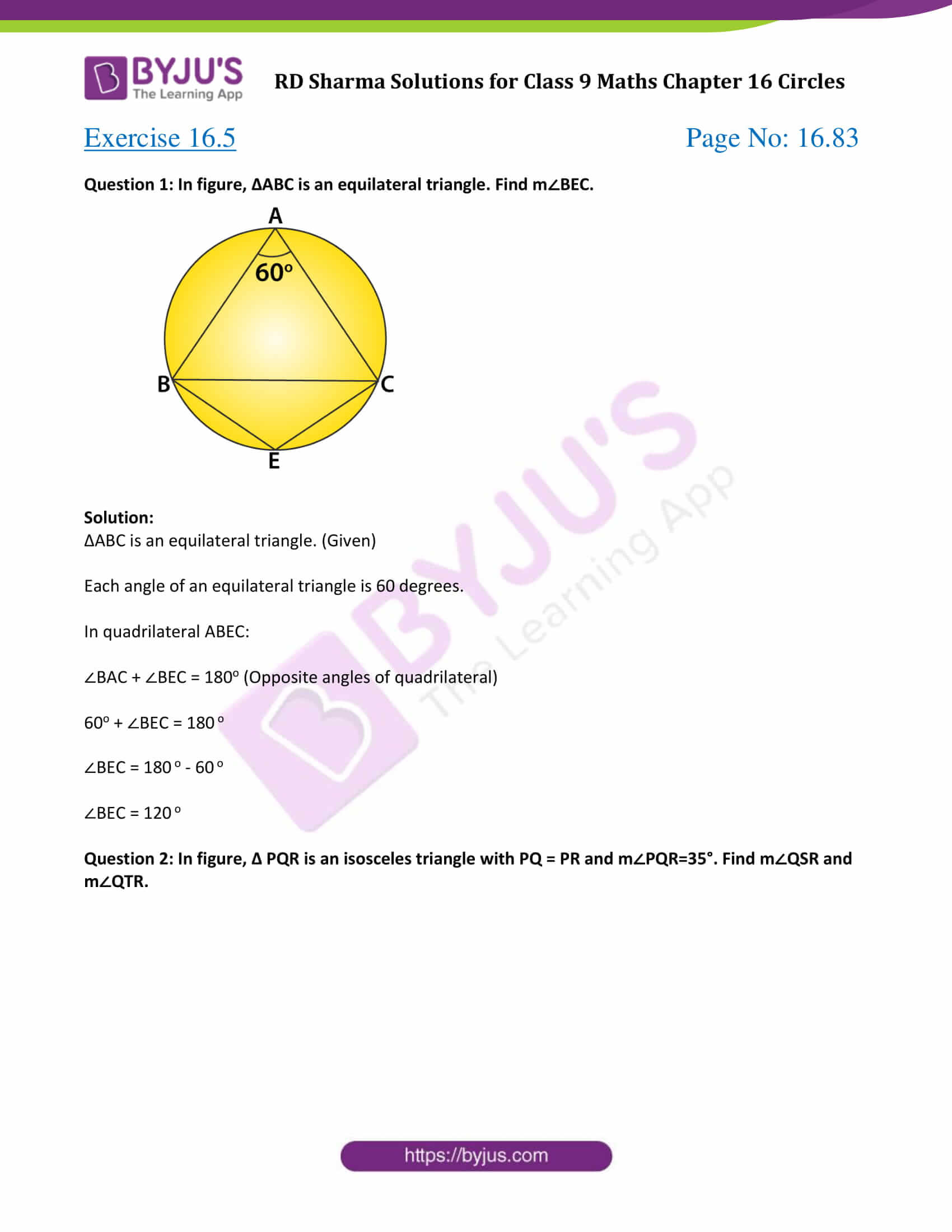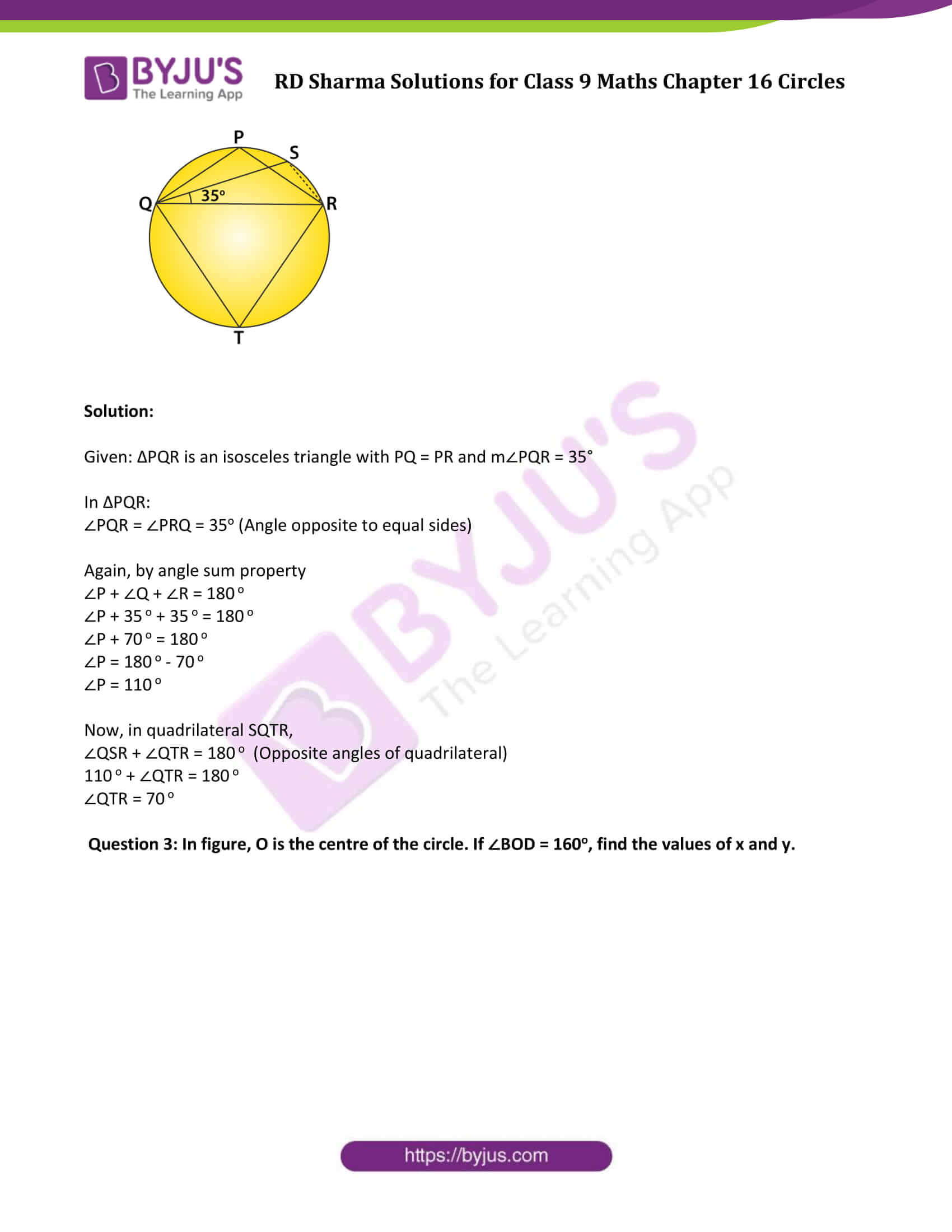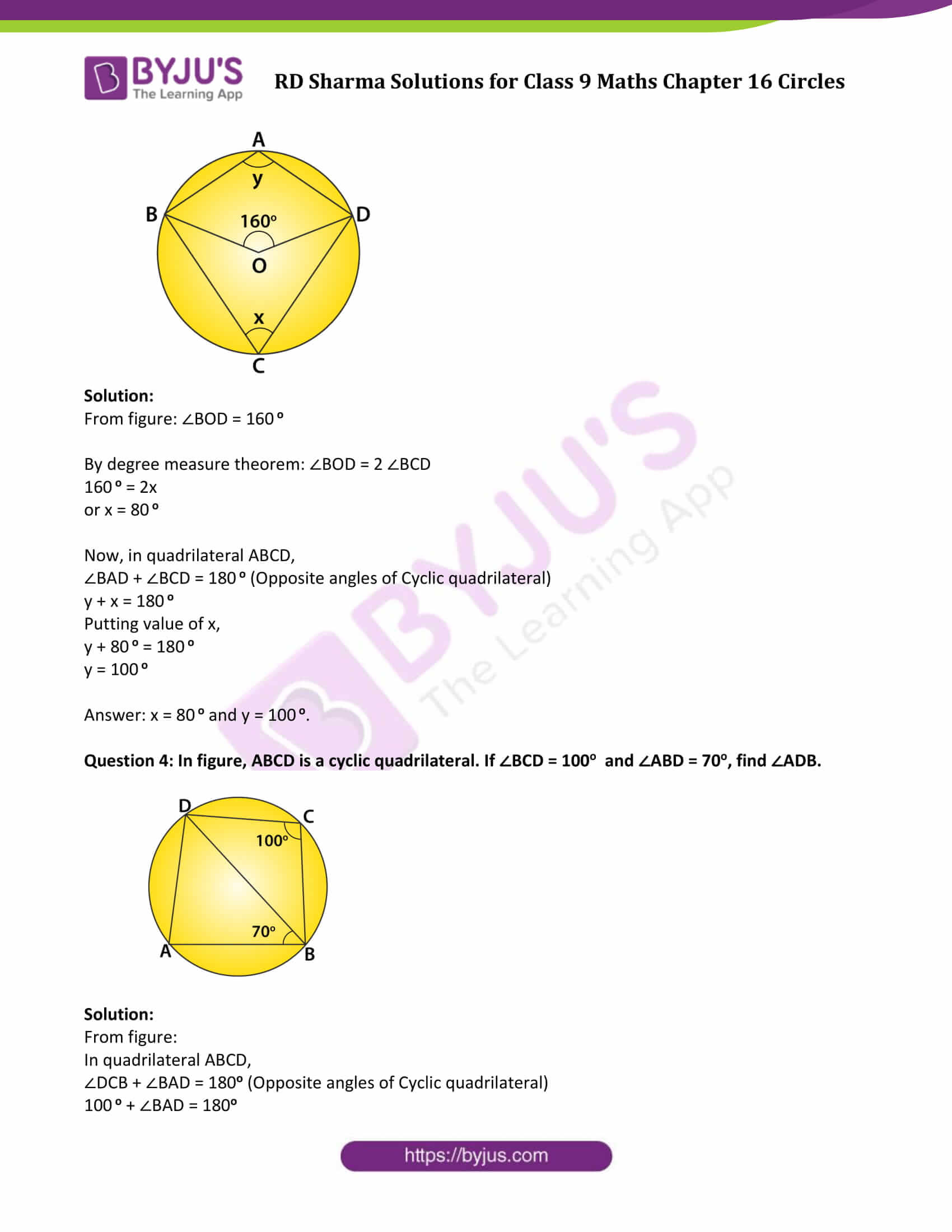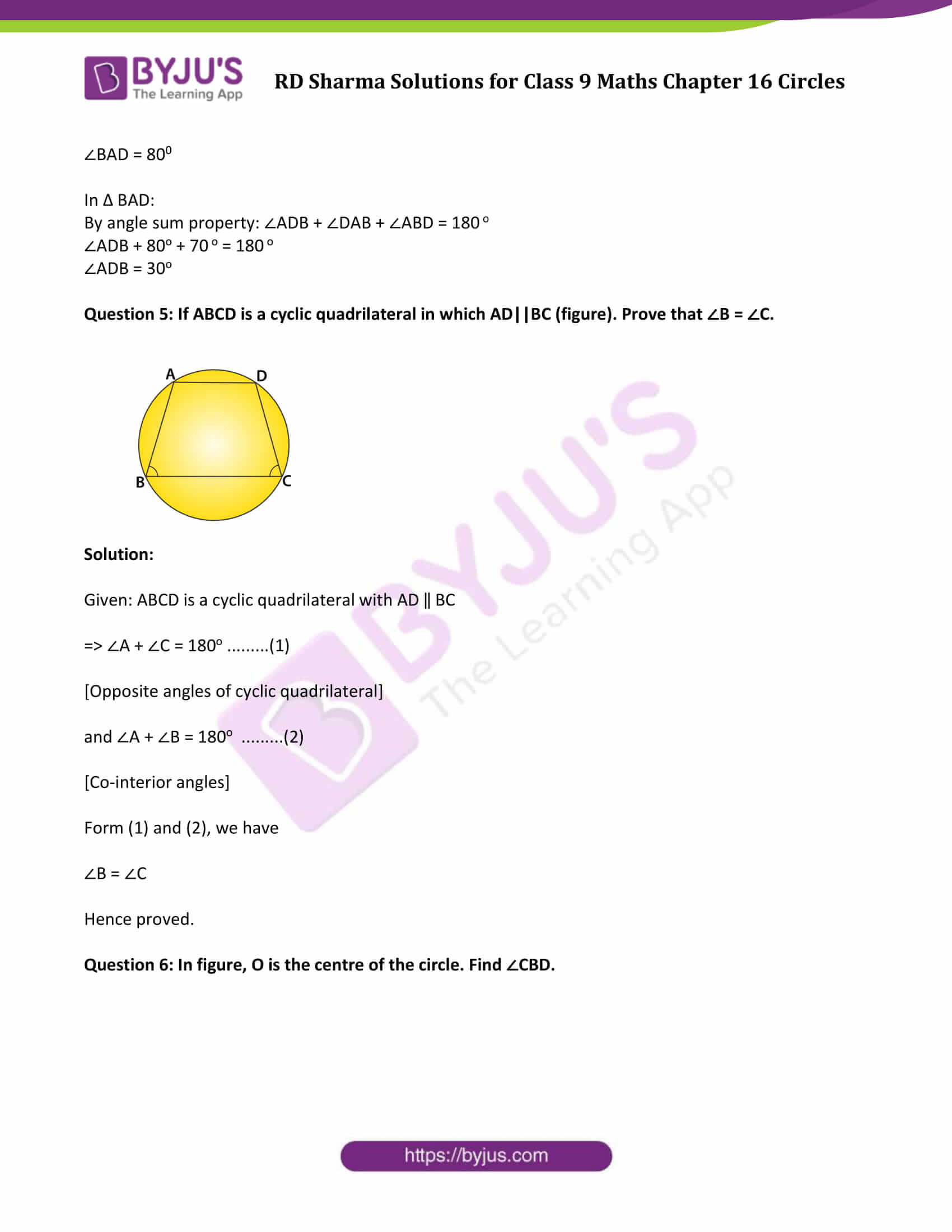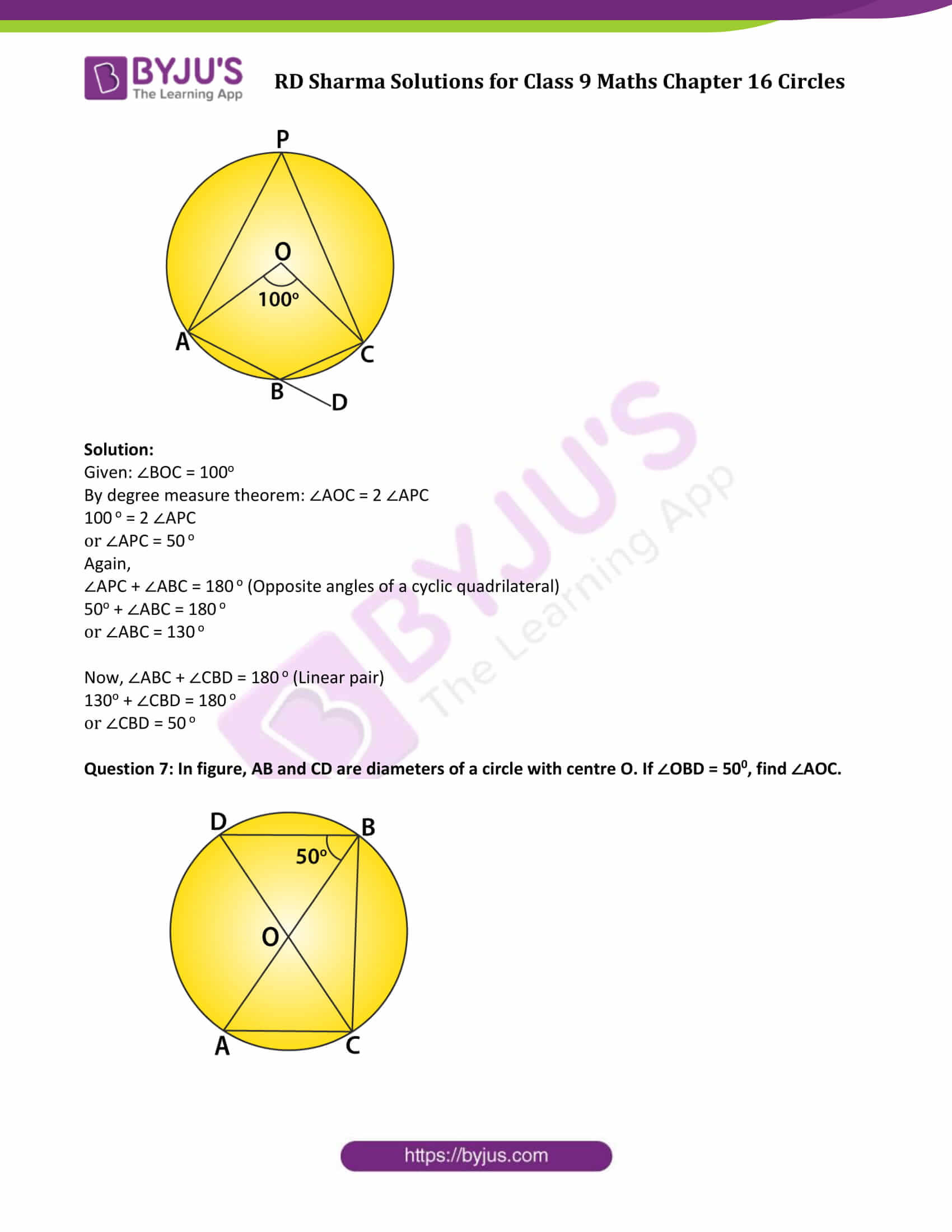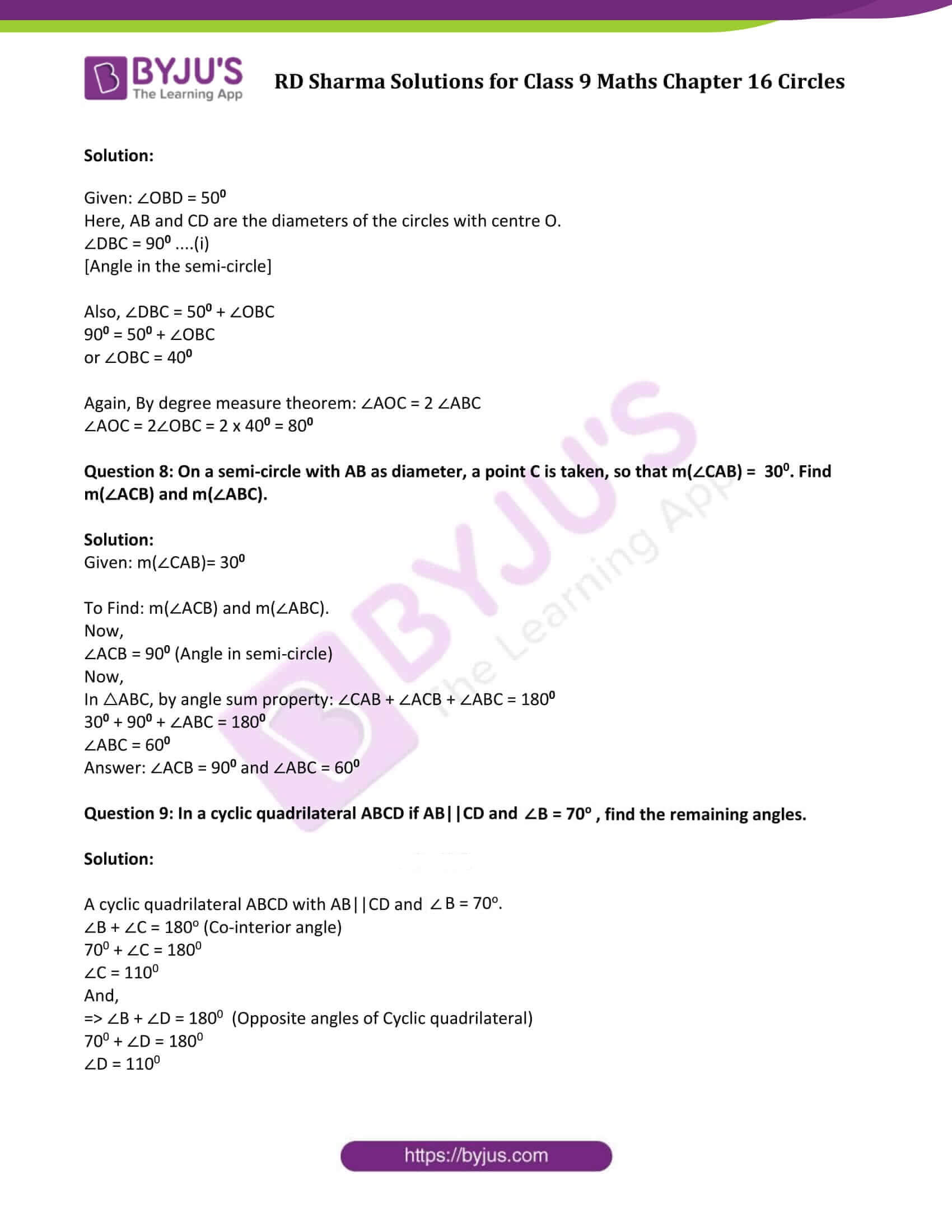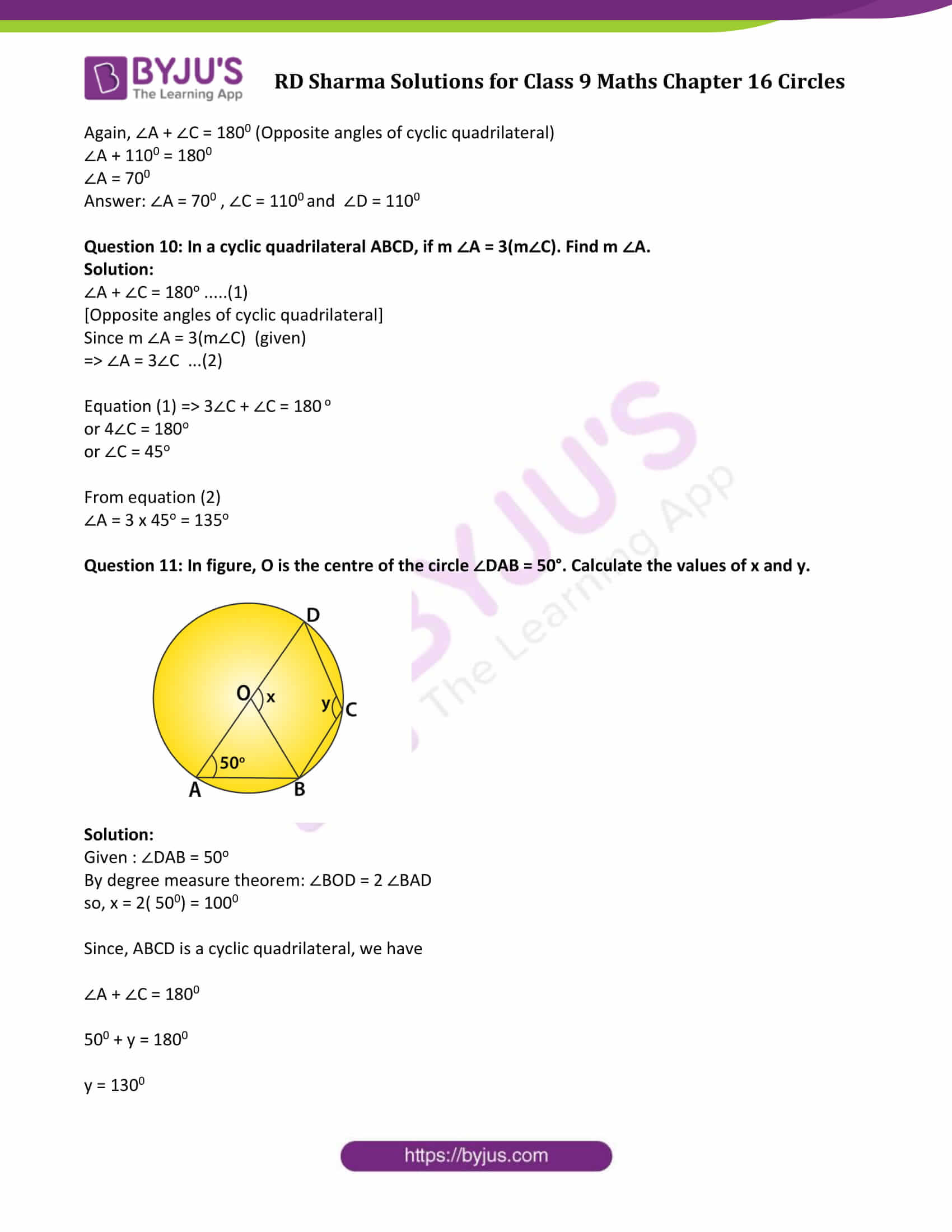### Access Answers to Maths RD Sharma Solutions for Class 9 Chapter 16 Circles Exercise 16.5 Page number 16.83

Question 1: In figure, ΔABC is an equilateral triangle. Find m∠BEC.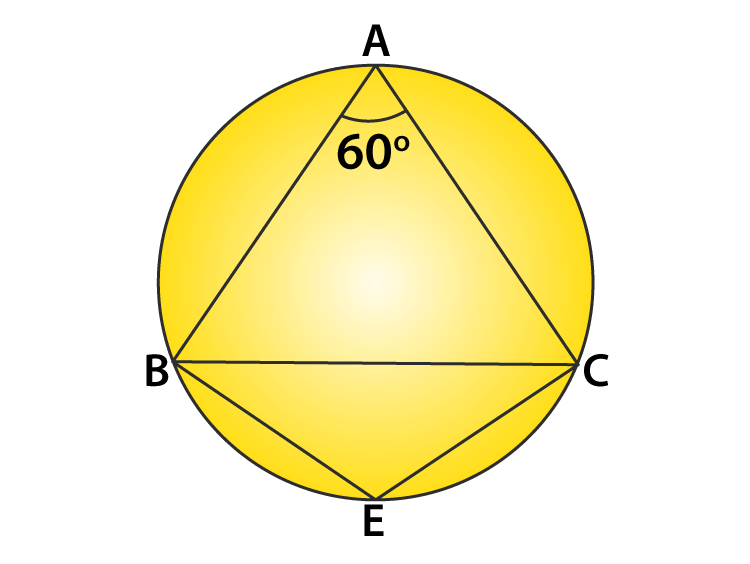Solution:

ΔABC is an equilateral triangle. (Given)

Each angle of an equilateral triangle is 60 degrees.

∠BAC + ∠BEC = 180o (Opposite angles of quadrilateral)

60o + ∠BEC = 180 o

∠BEC = 180 o – 60 o

∠BEC = 120 o

Question 2: In figure, Δ PQR is an isosceles triangle with PQ = PR and m∠PQR=35°. Find m∠QSR and m∠QTR.Solution:

Given: ΔPQR is an isosceles triangle with PQ = PR and m∠PQR = 35°

In ΔPQR:

∠PQR = ∠PRQ = 35o (Angle opposite to equal sides)

Again, by angle sum property

∠P + ∠Q + ∠R = 180 o

∠P + 35 o + 35 o = 180 o

∠P + 70 o = 180 o

∠P = 180 o – 70 o

∠P = 110 o

∠QSR + ∠QTR = 180 o (Opposite angles of quadrilateral)

110 o + ∠QTR = 180 o

∠QTR = 70 o

Question 3: In figure, O is the centre of the circle. If ∠BOD = 160o, find the values of x and y.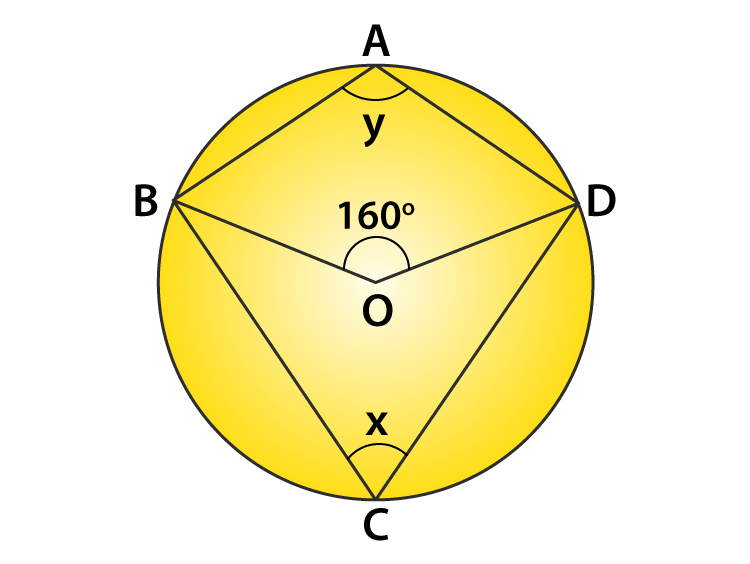Solution:

From figure: ∠BOD = 160 o

By degree measure theorem: ∠BOD = 2 ∠BCD

160 o = 2x

or x = 80 o

y + x = 180 o

Putting value of x,

y + 80 o = 180 o

y = 100 o

Answer: x = 80 o and y = 100 o.

Question 4: In figure, ABCD is a cyclic quadrilateral. If ∠BCD = 100o and ∠ABD = 70o, find ∠ADB.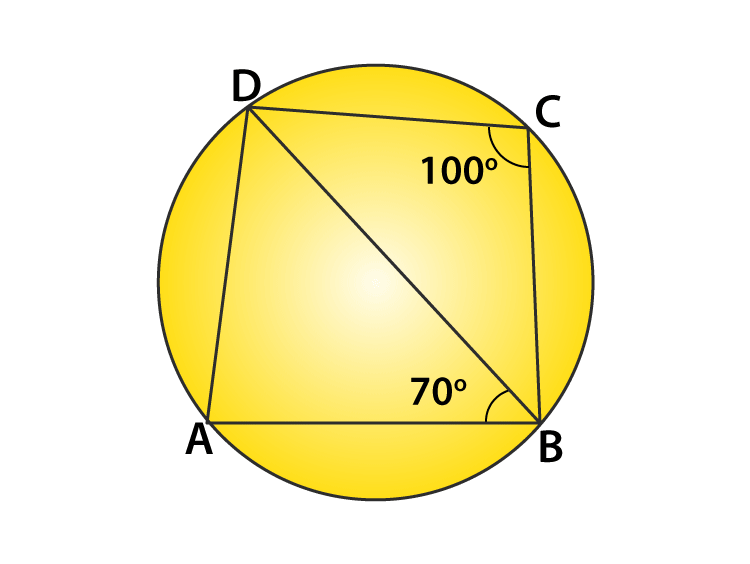Solution:

From figure:

100 o + ∠BAD = 180o

By angle sum property: ∠ADB + ∠DAB + ∠ABD = 180 o

∠ADB + 80o + 70 o = 180 o

Question 5: If ABCD is a cyclic quadrilateral in which AD||BC (figure). Prove that ∠B = ∠C.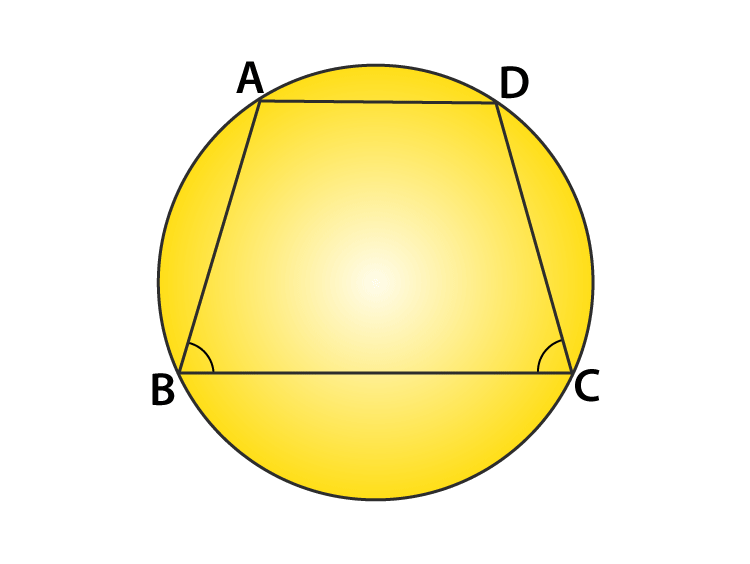Solution:

=> ∠A + ∠C = 180o ………(1)

and ∠A + ∠B = 180o ………(2)

[Co-interior angles]

Form (1) and (2), we have

∠B = ∠C

Hence proved.

Question 6: In figure, O is the centre of the circle. Find ∠CBD.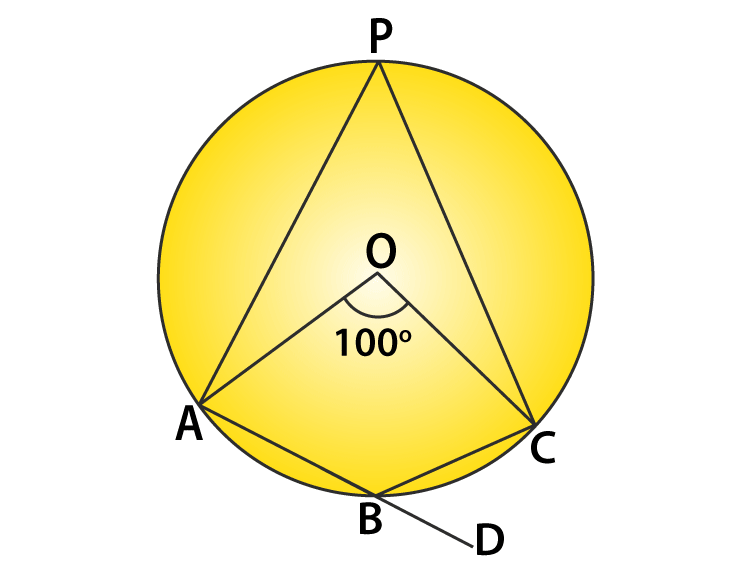Solution:

Given: ∠BOC = 100o

By degree measure theorem: ∠AOC = 2 ∠APC

100 o = 2 ∠APC

or ∠APC = 50 o

Again,

∠APC + ∠ABC = 180 o (Opposite angles of a cyclic quadrilateral)

50o + ∠ABC = 180 o

or ∠ABC = 130 o

Now, ∠ABC + ∠CBD = 180 o (Linear pair)

130o + ∠CBD = 180 o

or ∠CBD = 50 o

Question 7: In figure, AB and CD are diameters of a circle with centre O. If ∠OBD = 500, find ∠AOC.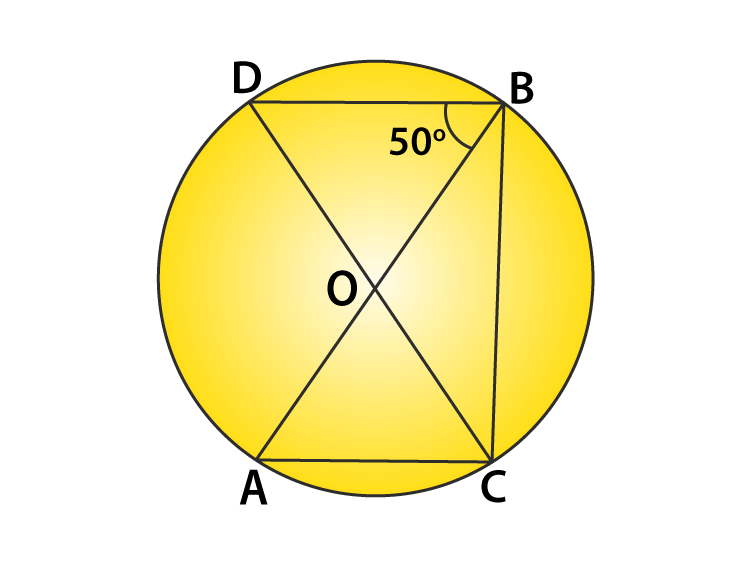Solution:

Given: ∠OBD = 500

Here, AB and CD are the diameters of the circles with centre O.

∠DBC = 900 ….(i)

[Angle in the semi-circle]

Also, ∠DBC = 500 + ∠OBC

900 = 500 + ∠OBC

or ∠OBC = 400

Again, By degree measure theorem: ∠AOC = 2 ∠ABC

∠AOC = 2∠OBC = 2 x 400 = 800

Question 8: On a semi-circle with AB as diameter, a point C is taken, so that m(∠CAB) = 300. Find m(∠ACB) and m(∠ABC).

Solution:

Given: m(∠CAB)= 300

To Find: m(∠ACB) and m(∠ABC).

Now,

∠ACB = 900 (Angle in semi-circle)

Now,

In △ABC, by angle sum property: ∠CAB + ∠ACB + ∠ABC = 1800

300 + 900 + ∠ABC = 1800

∠ABC = 600

Answer: ∠ACB = 900 and ∠ABC = 600

Question 9: In a cyclic quadrilateral ABCD if AB||CD and ∠B = 70o , find the remaining angles.

Solution:

A cyclic quadrilateral ABCD with AB||CD and ∠B = 70o.

∠B + ∠C = 180o (Co-interior angle)

700 + ∠C = 1800

∠C = 1100

And,

=> ∠B + ∠D = 1800 (Opposite angles of Cyclic quadrilateral)

700 + ∠D = 1800

∠D = 1100

Again, ∠A + ∠C = 1800 (Opposite angles of cyclic quadrilateral)

∠A + 1100 = 1800

∠A = 700

Answer: ∠A = 700 , ∠C = 1100 and ∠D = 1100

Question 10: In a cyclic quadrilateral ABCD, if m ∠A = 3(m∠C). Find m ∠A.
Solution:

∠A + ∠C = 180o …..(1)

Since m ∠A = 3(m∠C) (given)

=> ∠A = 3∠C …(2)

Equation (1) => 3∠C + ∠C = 180 o

or 4∠C = 180o

or ∠C = 45o

From equation (2)

∠A = 3 x 45o = 135o

Question 11: In figure, O is the centre of the circle ∠DAB = 50°. Calculate the values of x and y.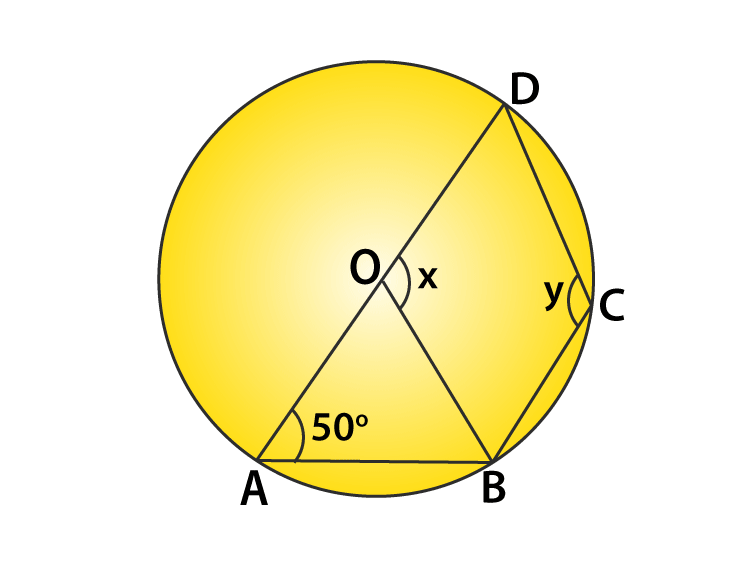Solution:

Given : ∠DAB = 50o

By degree measure theorem: ∠BOD = 2 ∠BAD

so, x = 2( 500) = 1000

Since, ABCD is a cyclic quadrilateral, we have

∠A + ∠C = 1800

500 + y = 1800

y = 1300

## RD Sharma Solutions for Class 9 Maths Chapter 16 Circles Exercise 16.5

RD Sharma Solutions Class 9 Maths Chapter 16 Circles Exercise 16.5 is based on the following topics and subtopics: